Next: Gulf of Mexico example Up: Multiple attenuation: Theory and Previous: Testing the Spitz approximation

# Adaptive vs. Pattern based subtraction

The goal of this section is to compare the pattern-based method with the more conventional adaptive subtraction on a synthetic dataset provided by BP. These two methods have very different properties. In one hand, adaptive subtraction assumes implicitly that the signal has minimum energy. In addition, the separation is very fast because the matching filters and the primaries are usually estimated simultaneously with Wiener-Levinson type methods. On the other hand, the proposed approach assumes that the signal and noise have different patterns. Unfortunately, high dimension filters for primaries and multiples need to be estimated first before starting the separation, which adds to the total cost of the method. Therefore, the pattern-based approach can be much slower than adaptive subtraction.

Computing considerations aside, the two methods can perform very differently according to the geological setting. Here, both are tested in a salt environment with a synthetic dataset. This dataset was primarily designed to conduct blind tests for velocity estimation methods. Consequently, no structural information is known. The adaptive subtraction technique used in this section is based on the estimation of 2-D, time/space varying matching filters Rickett et al. (2001). The filters are computed for one shot gather at a time. With the pattern-based approach, 3-D filters are used for the multiple attenuation. Ideally, 3-D filters should be also used with adaptive subtraction. However, matching filters are generally not estimated that way.

The multiple model is computed with SRMP. Therefore, only multiples bouncing at least once at the water surface will be modeled and subtracted. The synthetic model has an offset spacing of 12.5 m and a shot separation of 50 m. To make the multiple prediction work, the offset axis is sampled down to 50 m. Figureshows one constant offset section from -15,000 m to +15,000 m with primaries and multiples. This section of the dataset is particularly interesting because of the diffractions visible throughout. A possible interpretation of these diffractions is the presence of salt bodies with a rugose top (similar to what we see with the Sigsbee2B dataset). The multiple model is shown in Figurefor the same offset. DT points to diffraction tails where the model is not properly rendering the multiples in the data. Figureillustrates on a zero-offset example why diffractions are difficult to predict. The main reasons are that far offset data are not recorded and/or the scatter points are out of plane. Besides these few imperfections, the model looks very faithful to the actual multiples.

 scatter Figure 10 Illustration of a pegleg multiple with a bounce on a scatter point recorded at zero-offset. These events are difficult to model because of the lack of far and/or crossline offsets.The result of adaptive subtraction is shown for one offset section in Figureand the result of pattern-based subtraction is shown in Figure. The adaptive subtraction is doing a decent job everywhere. However, some multiples are still visible. For example, '1' in Figurepoints to a location where multiples overlap with primaries and are not attenuated. In contrast, the pattern-based subtraction (i.e., Figure) seems to do a better job attenuating these events. The same is true for arrows '2' and '5'. The diffracted multiples (arrows '3' and '4') are also attenuated more effectively with the pattern-based approach.

Because no velocity analysis was conducted with this dataset, no stacks are presented. Alternatively, close-ups of constant offset sections are shown to illustrate strengths and weaknesses of the two different approaches. Figureshows a comparison between the input data, the multiple model, the estimated primaries with the adaptive subtraction and the estimated primaries with the pattern-based technique. The offset is 700 m. As shown by the arrows, the pattern-based method performs generally better. The same conclusions hold in Figure. Note in Figureb aliasing artifacts due to the coarse sampling of the offset axis for the multiple prediction van Dedem (2002).

Sometimes, it can be rather difficult to see if multiples are removed or not by simply looking at 2-D planes. Figurec shows one event at '2' that seems to be a primary. However, by looking at the shot gathers (not shown here), it appears that this event is a multiple that the pattern-based approach was able to attenuate.

One shortcoming of the pattern-recognition technique is that it relies on the Spitz approximation to provide a signal model if nothing else is available. By construction, the signal and noise filters will span different components of the data space. Therefore, the estimated primaries and multiples are uncorrelated. This simple fact suggests that with the Spitz approximation, higher dimension filters are preferred because primaries and multiples have fewer chances to look similar.

Figureshows an example where primaries are damaged by the pattern-based method. For instance in Figurea, we see at '2' a primary that is attenuated by the PEFs (Figured) but well preserved by the adaptive subtraction (Figurec). Here the primaries and multiples (Figureb) exhibit similar patterns and the signal may have minimum energy. Using the Spitz approximation, event '2' is identified as noise and removed as such. For event '3', it is quite difficult to say if multiples are removed in Figured or if primaries are preserved in Figurec. Looking at the corresponding shot gathers did not help to make a decision because the multiples are very strong. Event '4' is preserved with the adaptive subtraction and '1' and '5' are well recovered with the pattern-based approach.

This synthetic example indicates that the pattern-based approach tend to attenuate the multiples more accurately than adaptive subtraction when the multiples are not correlated with the primaries. This illustrates that higher dimensions filters should be preferred to discriminate the noise and signal more effectively. The next section shows how the pattern-based approach performs on a field dataset from the Gulf of Mexico.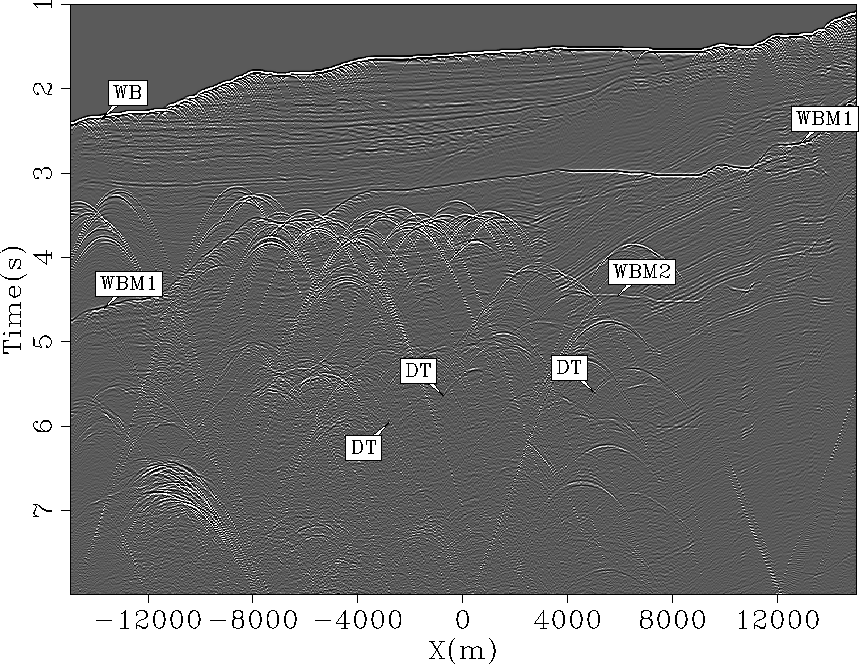data
Figure 11
Constant offset section (h=500 m) of the data with multiples. DTs point to the tails of diffracted multiples. These pegleg multiples bounce once on the seafloor and once on the scater. Arrow WB shows the water bottom, WBM1 the first order surface-related multiple, and WBM2 the second order surface-related multiple.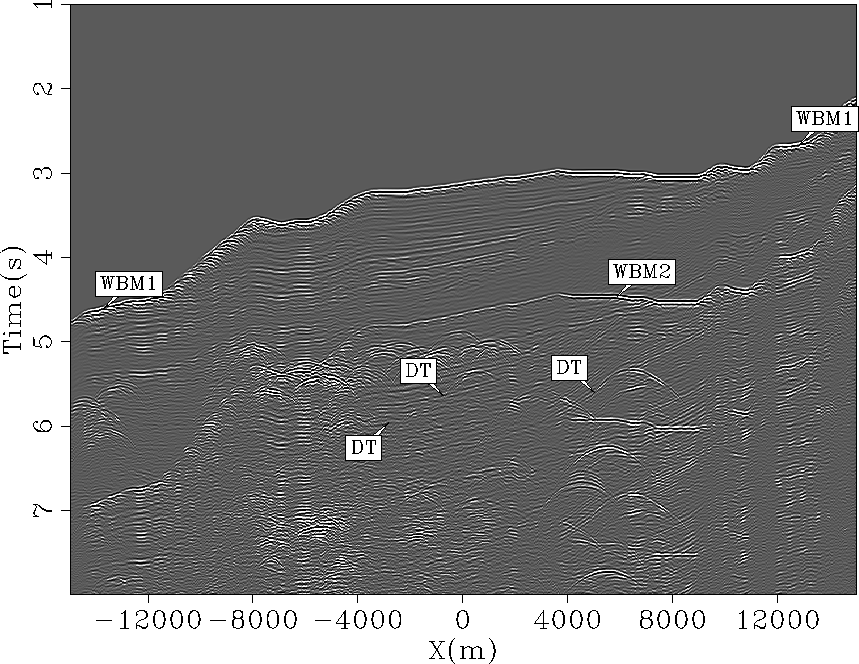mult
Figure 12
Constant offset section (h=550 m) of the estimated multiples. The multiples are accurately modeled except for the diffracted multiples, shown as DT, for which the limited range of offsets and number of shots hamper any attempt at modeling the diffraction tails. Arrow WBM1 shows the first order surface-related multiple, and WBM2 the second order surface-related multiple.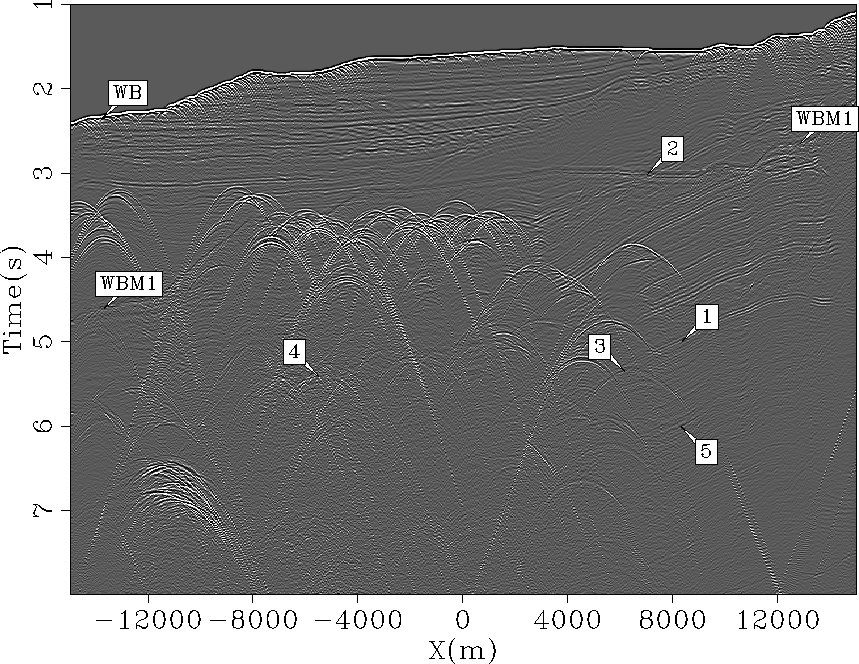dsign
Figure 13
Constant offset section (h=550 m) of the estimated primaries with adaptive subtraction. The arrows point to locations where multiples are still present. Arrow WBM1 shows the remaining energy for the first order surface-related multiple.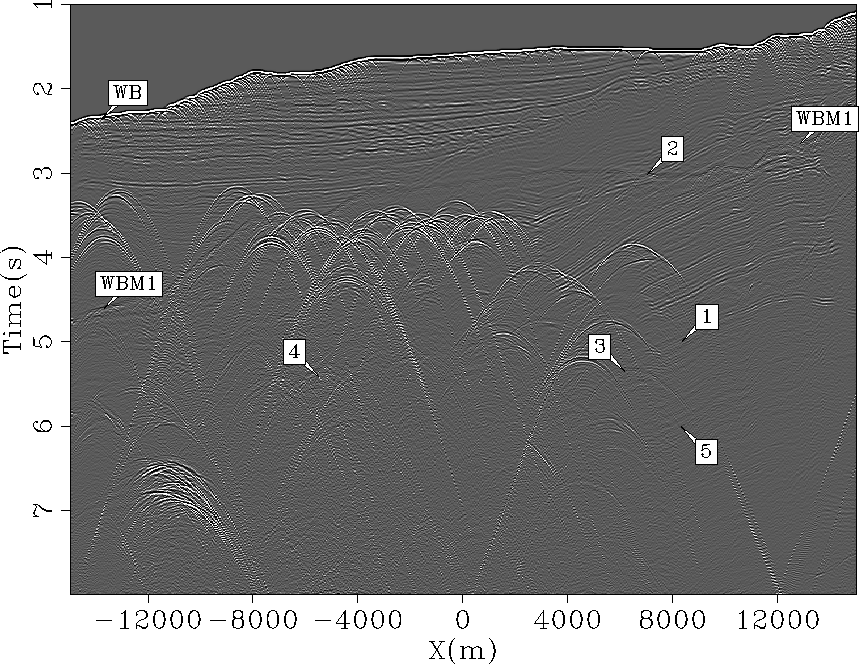sign
Figure 14
constant offset section (h=550 m) of the estimated primaries with pattern recognition. Multiples are better attenuated than in Figure. Arrow WBM1 shows the remaining energy for the first order surface-related multiple.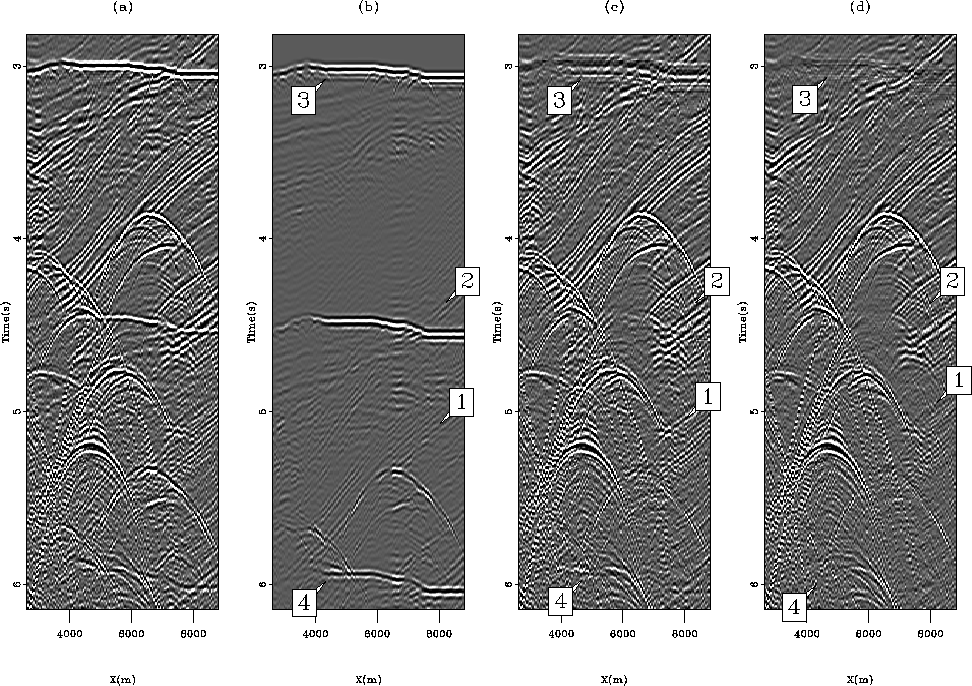compwin1
Figure 15
Constant offset sections (h=700 m) for (a) the input data, (b) the multiple model, (c) the estimated primaries with adaptive subtraction, and (d) estimated primaries with the pattern-based approach. Arrows point to locations where the pattern-based approach attenuates multiples significantly better than the adaptive subtraction.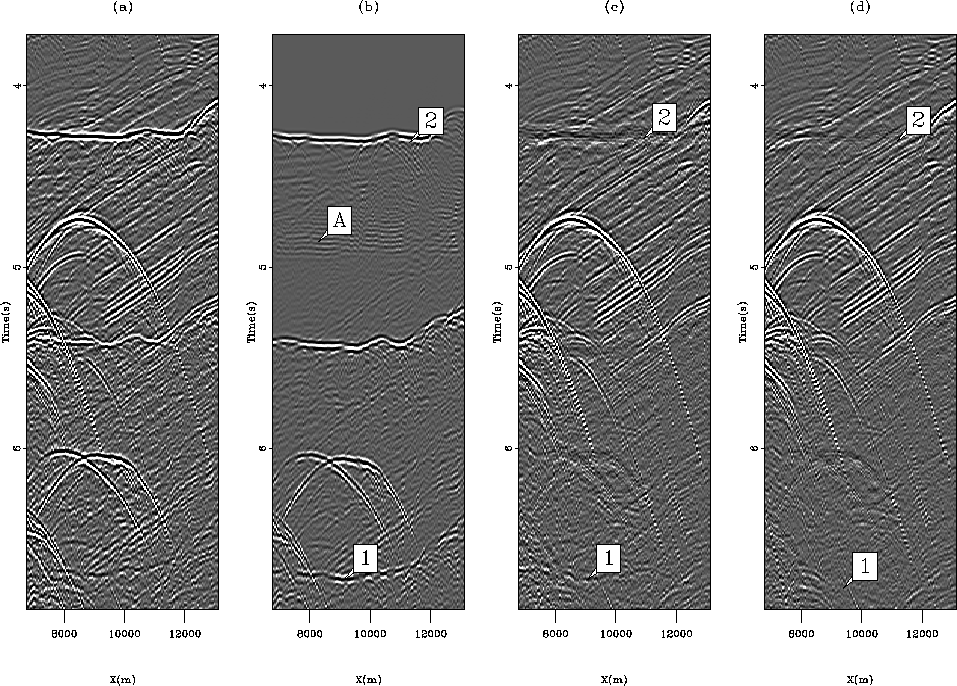compwin6
Figure 16
Constant offset sections (h=4550 m) for (a) the input data, (b) the multiple model, (c) the estimated primaries with adaptive subtraction, and (d) estimated primaries with the pattern-based approach. Arrow A points to aliasing effects due to the offset sampling of the shot gathers. The pattern-based approach attenuates the multiples better than the adaptive subtraction in '1' and '2'.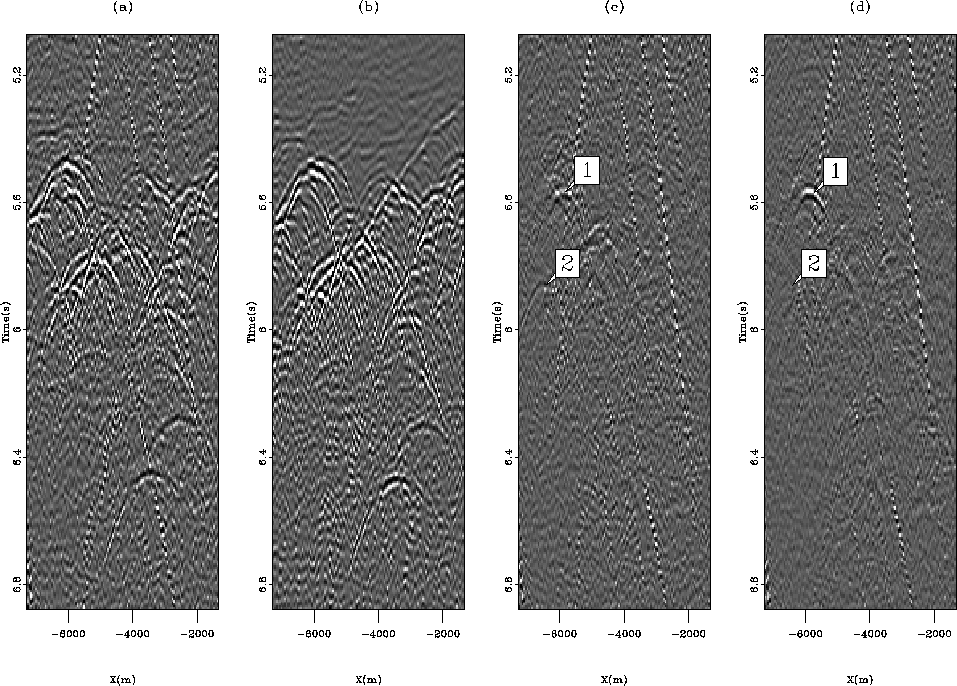compwin8
Figure 17
Constant offset sections (h=3300 m) for (a) the input data, (b) the multiple model, (c) the estimated primaries with adaptive subtraction, and (d) estimated primaries with the pattern-based approach. '1' points to a primary that the pattern-recognition preserves very well. '2' points to an event that is attenuated with the pattern-based approach but not with the adaptive subtraction in (c). Though not shown here, a close inspection of the corresponding shot gathers suggests that '2' is actually a multiple.compwin12
Figure 18
Constant offset sections (h=5050 m) for (a) the input data, (b) the multiple model, (c) the estimated primaries with adaptive subtraction, and (d) estimated primaries with the pattern-based approach. '1' and '5' show events better preserved with the pattern-based method. '2' and '1' are better recovered with the adaptive subtraction. '4' seems to point to a primary that the adaptive subtraction is able to save. Because the area is contaminated with strong multiples, it is difficult to know without a stratigraphic model if '3' is a primary or not.Next: Gulf of Mexico example Up: Multiple attenuation: Theory and Previous: Testing the Spitz approximation
Stanford Exploration Project
5/5/2005